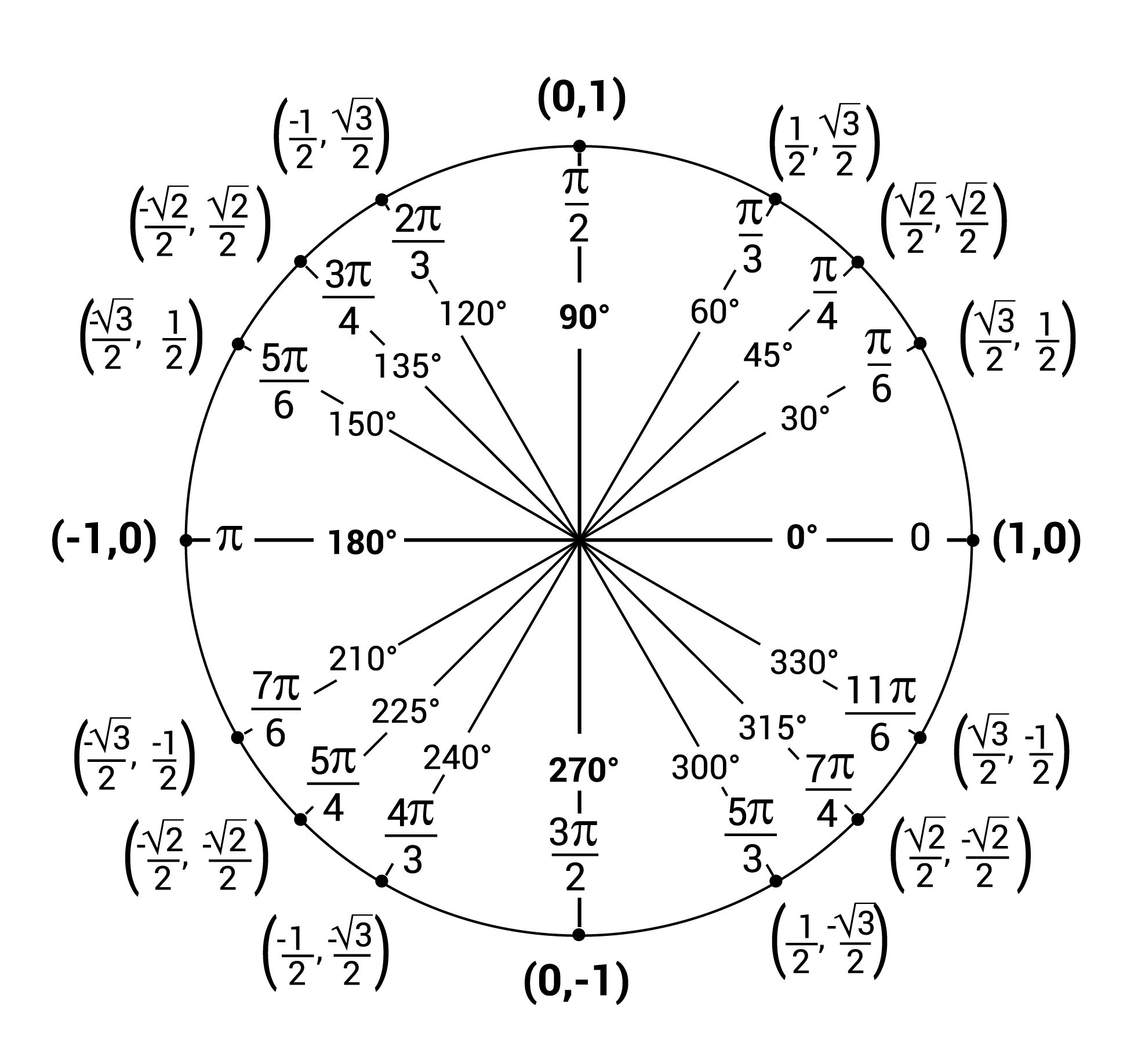The unit circle is a fundamental concept in trigonometry that provides a geometric interpretation of the trigonometric functions sine and cosine. In this article, we will explore the unit circle, understand what radians are, and learn how the points on the unit circle correspond to the sine and cosine of angles.

## What is the Unit Circle?

The unit circle is a circle with a radius of 1 unit, centered at the origin (0,0) of a Cartesian coordinate system. The equation of the unit circle is $$x^2 + y^2 = 1$$.Radians are a unit of angular measure used in mathematics. One radian is defined as the angle subtended at the center of a circle by an arc whose length is equal to the radius of the circle. In simpler terms, if you wrap the radius of a circle around its circumference, the angle formed at the center is 1 radian.

There are $$2\pi$$ radians in a complete circle, which is equivalent to 360 degrees. To convert from degrees to radians, you can use the formula:

$\text{Radians} = \text{Degrees} \times \frac{\pi}{180}$## Sine and Cosine on the Unit Circle

Any point $$P$$ on the unit circle can be represented by coordinates $$(x, y)$$, where $$x = \cos(\theta)$$ and $$y = \sin(\theta)$$. Here, $$\theta$$ is the angle in radians formed by the line segment joining the origin and point $$P$$ with the positive x-axis.

For example, if $$\theta = \frac{\pi}{4}$$ radians (or 45 degrees), then the coordinates of point $$P$$ would be $$\left(\frac{\sqrt{2}}{2}, \frac{\sqrt{2}}{2}\right)$$.

This geometric interpretation allows us to understand the periodic nature of sine and cosine functions and provides a way to visualize these functions beyond the scope of right-angled triangles.

## Conclusion

The unit circle is a powerful tool for understanding the properties of trigonometric functions. By using radians as our unit of angular measure, we can easily relate the geometry of the circle to the algebra of trigonometry.